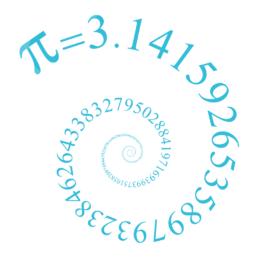Bournemouth Maths Tutor Barney M-TQuote of the week: DIFFERENTIATION IS A SKILL. INTEGRATION IS AN ART.

I was delighted to find this quote at the start of Seán M. Stewart‘s book “How to Integrate it“.One of the main differences between GCSE and A-level maths is the introduction of calculus. This branch of maths makes sense of the infinitely small, allowing us to deal with quantities that vary continuously (often over time)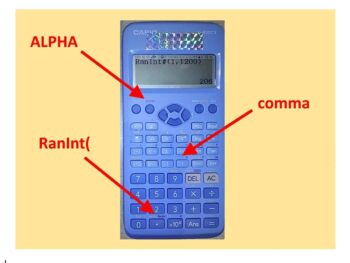TOP TEN SAMPLING METHODS

A quick reminder: if there are 1200 students at our school, it would be very time-consuming (if not impossible) to interview them all for your Sociology project. Here the population is everyone at the school, and interviewing them all would be called taking a census. Instead, it’s more practical to interview just a selection –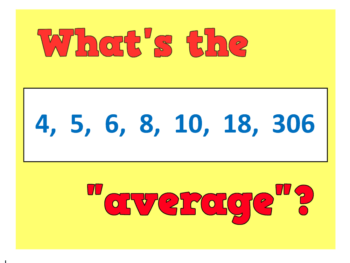WHAT IS THE “AVERAGE” IN MATHS?

An average is simply a way of picking out a typical value from a set of objects (usually numbers). There are infinitely many different ways to pick a “typical value”, but here are some of my favourites: Midrange average: The simplest of the lot: just calculate the number that is half way between the smallest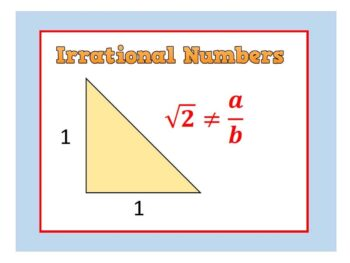Strange but True: IRRATIONAL NUMBERS

The first numbers we discover in childhood are the Natural Numbers: 1, 2, 3, … Next come the Integers (whole numbers) – which also include Zero and the negatives: … -3, -2, -1, 0, 1, 2, 3, … Next up: it’s fractions such as: 0.5, 2.90909… , $-\Large{5}\frac{7}{8}$ Numbers like these are said to be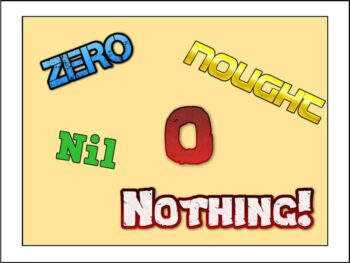(or: Much ado about Nothing) Zero doesn’t actually exist. Think about it: by definition it isn’t anything, it’s nothing!! Even so, here are 5 facts every mathematician should know about Zero: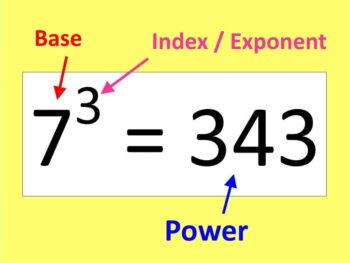WORD OF THE WEEK: INDEX

INDEX (plural indices): you can see these in expressions like $5^2 = 25$. The 5 is called the BASE, the 2 is called the EXPONENT or INDEX, and the 25 is called a power (in this case it’s a power of 5).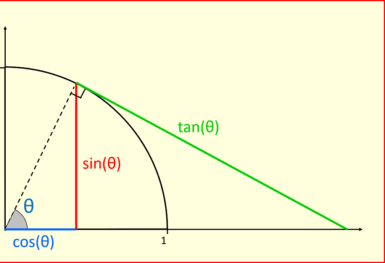WORD OF THE WEEK: TRIGONOMETRY

TRIGONOMETRY is the branch of maths to do with sin, cos and tan (find them on your calculator). Literally it means “measuring triangles” – so you can use these three functions to find unknown lengths and angles. Sin is short for “sine” – which is why it should be pronounced “sign” not “sinn”. Cos and